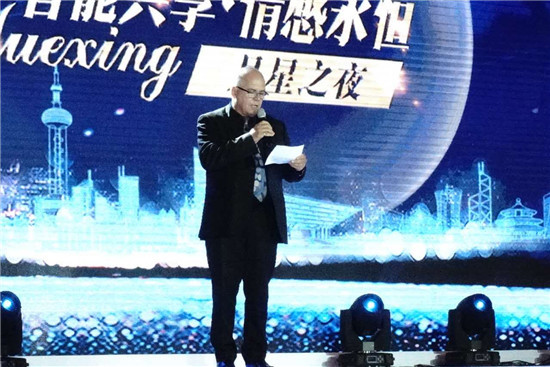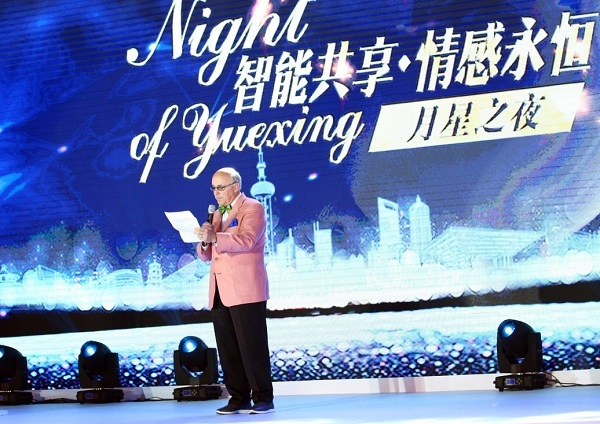|

# 智能共享·情感永恒 月星家居智慧商城重磅发布

9月12日，月星家居在上海澳门路店月星家居茂，举办的主题为：“智能共享·情感永恒”的“月星之夜”活动，已经圆满落幕。

此次活动，表明月星正努力向月星的厂商和经销商提供更优质的服务，并用最先进的智能化技术，打造一个连接着月星、厂商、经销商、终端消费者的高效共享产业生态平台。

活动现场，全国工商联家具装饰业商会会长、月星集团董事局主席丁佐宏根据多年的经验和摸索，提出月星家居建立十大中心的构想【十大中心：家居博览中心、智慧商城中心、生活中心、设计中心、文化中心、检测中心、服务中心、保税中心、信息中心、科创中心】，得到现场嘉宾的广泛认可。现场嘉宾云集，行业大佬各陈己见。

月星家居是月星集团下面的一家连锁型的家居建材一站式购物市场，从1988年创立至今的19年时间里，已经在全国15个省市都设有分市场。月星也从家居制造起家后，涉足家居、商业、制造等多行业领域，发展成一家业务多元化的大型集团。

为了此次月星之夜活动的圆满召开，月星家居邀请了众多的行业大佬，并在活动中发表了对市场的看法。

中国黑龙江省黑河市委副书记姚志波上台发言，他表示随着一带一路计划的推进，黑龙江地处边界，正在搭建跨境贸易平台，这次到月星考察，发现月星的发展模式正是适合跨境贸易的项目，相信未来月星的发展会越来越好，将走出国门，走进俄罗斯。澳大利亚昆士兰州耶蓬市市长比尔.路德维格上台发言。他表示月星之夜是一次非凡之旅，中国家居行业以精良的品质和创新的精神，创造了目前中国家居业的繁荣。而月星环球港则是丁佐宏主席非凡创造力的成果。美国家具设计师学会CEO John Conrad上台发言。月星是一个交融的平台，求同存异，不同的品牌、企业设计师带来了不同的产品，带来更多本土化的东西，但是却拥有共同的理念。智慧商城，改变行业的新突破

月星家居月星之夜活动现场，月星集团常务副总裁顾春峰先生带来关于“智能共享平台”的演讲。他表示月星“智造百茂”正在得到稳步的实施。不仅是内涵式的提升，更进行了外延式的发展。月星的十大中心让智能更贴近消费者，在活动中推出的“月星家居智慧商场”和一系列有建设性的发展方针，使十大中心的构思得到落实，并得到现场嘉宾的认可。尤其是“月星家居智慧商场”的推出，更是改变市影响整个家居行业大动作。

月星家居智慧商城是月星家居集团电商团队对O2O新零售模式的探索和尝试，它是一个创意与互联网思维结合的时代产物。为消费者、合作伙伴带来更多边际效益的同时，为线下的合作伙伴引流，增加消费粘度，提升企业的效益；通过数字化平台的规模化运作，降低合作伙伴的经营成本；通过数字化平台的大数据共享，促进上下游间的商业合作和产品交易。

月星家居智慧商城满足客户对模版化DIY家居设计和在家VR实景逛商场的需求。设置每天16小时的在线的商品导购和可以随时沟通的网上设计师免费提供软装咨询，以吸引聚集大量的网上精准客户。通过进店客户免费wi-fi上网、电子地图、微信扫价签购物、浏览商户和产品点评等技术上的支持，将线下客户转化成线上会员，通过“线上+线下+会员”模式完成带来会员和客户的极速增长。亮点不断，用创意营造现场气氛。

活动现场，除了大佬发言和智慧商城的发布，月星还邀请大家体验专为本次活动研发的关于智慧商城的H5创意小游戏，让大家在短暂休息的同时，体会到了月星电商团队的创意和对于新时代互联网新生代的理解和诠释。

此次活动中，月星电商还精心推出了高端科技游戏设备——登月舱。登月舱使用人体工学按摩椅和电竞主机外设。为广大的消费者在逛商场的闲暇之余，提供了一个可以休息娱乐的场所。目前已在上海环球港四楼、上海澳门路店月星家居茂一楼大厅安置，供广大的消费者使用。现场另外安排了网红主播亲自操作登月舱，给广大网友演示展示游戏体验。

“月星之夜”是一场家居行业的标杆性晚会，此次月星之夜，再一次用创意营造了完美的活动气氛，用智慧云端改变了市场，影响整个家居行业。尤其是此次推出的智慧商城，改变了国人对家居圈的理解和看法，改变所有消费者的消费思维和消费模式，最重要的是，智慧商城改变每一个人的家具购买方式，给所有人提供更为快捷，更为透明，更为真实，更为安全的家具购买渠道。

据悉“智能共享，情感永恒”是本次月星之夜的主题，也将会是未来整个家居集团的战略主题，它将如何诠释带给世人所知？让我们拭目以待！`声明：本文由入驻焦点开放平台的作者撰写，除焦点官方账号外，观点仅代表作者本人，不代表焦点立场错误信息举报电话： 400-099-0099，邮箱：jubao@vip.sohu.com，或点此进行意见反馈，或点此进行举报投诉。`A B C D E F G H J K L M N P Q R S T W X Y Z
A - B - C - D - E
• A
• 鞍山
• 安庆
• 安阳
• 安顺
• 安康
• 澳门
• B
• 北京
• 保定
• 包头
• 巴彦淖尔
• 本溪
• 蚌埠
• 亳州
• 滨州
• 北海
• 百色
• 巴中
• 毕节
• 保山
• 宝鸡
• 白银
• 巴州
• C
• 承德
• 沧州
• 长治
• 赤峰
• 朝阳
• 长春
• 常州
• 滁州
• 池州
• 长沙
• 常德
• 郴州
• 潮州
• 崇左
• 重庆
• 成都
• 楚雄
• 昌都
• 慈溪
• 常熟
• D
• 大同
• 大连
• 丹东
• 大庆
• 东营
• 德州
• 东莞
• 德阳
• 达州
• 大理
• 德宏
• 定西
• 儋州
• 东平
• E
• 鄂尔多斯
• 鄂州
• 恩施
F - G - H - I - J
• F
• 抚顺
• 阜新
• 阜阳
• 福州
• 抚州
• 佛山
• 防城港
• G
• 赣州
• 广州
• 桂林
• 贵港
• 广元
• 广安
• 贵阳
• 固原
• H
• 邯郸
• 衡水
• 呼和浩特
• 呼伦贝尔
• 葫芦岛
• 哈尔滨
• 黑河
• 淮安
• 杭州
• 湖州
• 合肥
• 淮南
• 淮北
• 黄山
• 菏泽
• 鹤壁
• 黄石
• 黄冈
• 衡阳
• 怀化
• 惠州
• 河源
• 贺州
• 河池
• 海口
• 红河
• 汉中
• 海东
• 怀来
• I
• J
• 晋中
• 锦州
• 吉林
• 鸡西
• 佳木斯
• 嘉兴
• 金华
• 景德镇
• 九江
• 吉安
• 济南
• 济宁
• 焦作
• 荆门
• 荆州
• 江门
• 揭阳
• 金昌
• 酒泉
• 嘉峪关
K - L - M - N - P
• K
• 开封
• 昆明
• 昆山
• L
• 廊坊
• 临汾
• 辽阳
• 连云港
• 丽水
• 六安
• 龙岩
• 莱芜
• 临沂
• 聊城
• 洛阳
• 漯河
• 娄底
• 柳州
• 来宾
• 泸州
• 乐山
• 六盘水
• 丽江
• 临沧
• 拉萨
• 林芝
• 兰州
• 陇南
• M
• 牡丹江
• 马鞍山
• 茂名
• 梅州
• 绵阳
• 眉山
• N
• 南京
• 南通
• 宁波
• 南平
• 宁德
• 南昌
• 南阳
• 南宁
• 内江
• 南充
• P
• 盘锦
• 莆田
• 平顶山
• 濮阳
• 攀枝花
• 普洱
• 平凉
Q - R - S - T - W
• Q
• 秦皇岛
• 齐齐哈尔
• 衢州
• 泉州
• 青岛
• 清远
• 钦州
• 黔南
• 曲靖
• 庆阳
• R
• 日照
• 日喀则
• S
• 石家庄
• 沈阳
• 双鸭山
• 绥化
• 上海
• 苏州
• 宿迁
• 绍兴
• 宿州
• 三明
• 上饶
• 三门峡
• 商丘
• 十堰
• 随州
• 邵阳
• 韶关
• 深圳
• 汕头
• 汕尾
• 三亚
• 三沙
• 遂宁
• 山南
• 商洛
• 石嘴山
• T
• 天津
• 唐山
• 太原
• 通辽
• 铁岭
• 泰州
• 台州
• 铜陵
• 泰安
• 铜仁
• 铜川
• 天水
• 天门
• W
• 乌海
• 乌兰察布
• 无锡
• 温州
• 芜湖
• 潍坊
• 威海
• 武汉
• 梧州
• 渭南
• 武威
• 吴忠
• 乌鲁木齐
X - Y - Z
• X
• 邢台
• 徐州
• 宣城
• 厦门
• 新乡
• 许昌
• 信阳
• 襄阳
• 孝感
• 咸宁
• 湘潭
• 湘西
• 西双版纳
• 西安
• 咸阳
• 西宁
• 仙桃
• 西昌
• Y
• 运城
• 营口
• 盐城
• 扬州
• 鹰潭
• 宜春
• 烟台
• 宜昌
• 岳阳
• 益阳
• 永州
• 阳江
• 云浮
• 玉林
• 宜宾
• 雅安
• 玉溪
• 延安
• 榆林
• 银川
• Z
• 张家口
• 镇江
• 舟山
• 漳州
• 淄博
• 枣庄
• 郑州
• 周口
• 驻马店
• 株洲
• 张家界
• 珠海
• 湛江
• 肇庆
• 中山
• 自贡
• 资阳
• 遵义
• 昭通
• 张掖
• 中卫

1室1厅1厨1卫1阳台

1
2
3
4
5

0
1
2

1

1

0
1
2
3报名成功，资料已提交审核A B C D E F G H J K L M N P Q R S T W X Y Z
A - B - C - D - E
• A
• 鞍山
• 安庆
• 安阳
• 安顺
• 安康
• 澳门
• B
• 北京
• 保定
• 包头
• 巴彦淖尔
• 本溪
• 蚌埠
• 亳州
• 滨州
• 北海
• 百色
• 巴中
• 毕节
• 保山
• 宝鸡
• 白银
• 巴州
• C
• 承德
• 沧州
• 长治
• 赤峰
• 朝阳
• 长春
• 常州
• 滁州
• 池州
• 长沙
• 常德
• 郴州
• 潮州
• 崇左
• 重庆
• 成都
• 楚雄
• 昌都
• 慈溪
• 常熟
• D
• 大同
• 大连
• 丹东
• 大庆
• 东营
• 德州
• 东莞
• 德阳
• 达州
• 大理
• 德宏
• 定西
• 儋州
• 东平
• E
• 鄂尔多斯
• 鄂州
• 恩施
F - G - H - I - J
• F
• 抚顺
• 阜新
• 阜阳
• 福州
• 抚州
• 佛山
• 防城港
• G
• 赣州
• 广州
• 桂林
• 贵港
• 广元
• 广安
• 贵阳
• 固原
• H
• 邯郸
• 衡水
• 呼和浩特
• 呼伦贝尔
• 葫芦岛
• 哈尔滨
• 黑河
• 淮安
• 杭州
• 湖州
• 合肥
• 淮南
• 淮北
• 黄山
• 菏泽
• 鹤壁
• 黄石
• 黄冈
• 衡阳
• 怀化
• 惠州
• 河源
• 贺州
• 河池
• 海口
• 红河
• 汉中
• 海东
• 怀来
• I
• J
• 晋中
• 锦州
• 吉林
• 鸡西
• 佳木斯
• 嘉兴
• 金华
• 景德镇
• 九江
• 吉安
• 济南
• 济宁
• 焦作
• 荆门
• 荆州
• 江门
• 揭阳
• 金昌
• 酒泉
• 嘉峪关
K - L - M - N - P
• K
• 开封
• 昆明
• 昆山
• L
• 廊坊
• 临汾
• 辽阳
• 连云港
• 丽水
• 六安
• 龙岩
• 莱芜
• 临沂
• 聊城
• 洛阳
• 漯河
• 娄底
• 柳州
• 来宾
• 泸州
• 乐山
• 六盘水
• 丽江
• 临沧
• 拉萨
• 林芝
• 兰州
• 陇南
• M
• 牡丹江
• 马鞍山
• 茂名
• 梅州
• 绵阳
• 眉山
• N
• 南京
• 南通
• 宁波
• 南平
• 宁德
• 南昌
• 南阳
• 南宁
• 内江
• 南充
• P
• 盘锦
• 莆田
• 平顶山
• 濮阳
• 攀枝花
• 普洱
• 平凉
Q - R - S - T - W
• Q
• 秦皇岛
• 齐齐哈尔
• 衢州
• 泉州
• 青岛
• 清远
• 钦州
• 黔南
• 曲靖
• 庆阳
• R
• 日照
• 日喀则
• S
• 石家庄
• 沈阳
• 双鸭山
• 绥化
• 上海
• 苏州
• 宿迁
• 绍兴
• 宿州
• 三明
• 上饶
• 三门峡
• 商丘
• 十堰
• 随州
• 邵阳
• 韶关
• 深圳
• 汕头
• 汕尾
• 三亚
• 三沙
• 遂宁
• 山南
• 商洛
• 石嘴山
• T
• 天津
• 唐山
• 太原
• 通辽
• 铁岭
• 泰州
• 台州
• 铜陵
• 泰安
• 铜仁
• 铜川
• 天水
• 天门
• W
• 乌海
• 乌兰察布
• 无锡
• 温州
• 芜湖
• 潍坊
• 威海
• 武汉
• 梧州
• 渭南
• 武威
• 吴忠
• 乌鲁木齐
X - Y - Z
• X
• 邢台
• 徐州
• 宣城
• 厦门
• 新乡
• 许昌
• 信阳
• 襄阳
• 孝感
• 咸宁
• 湘潭
• 湘西
• 西双版纳
• 西安
• 咸阳
• 西宁
• 仙桃
• 西昌
• Y
• 运城
• 营口
• 盐城
• 扬州
• 鹰潭
• 宜春
• 烟台
• 宜昌
• 岳阳
• 益阳
• 永州
• 阳江
• 云浮
• 玉林
• 宜宾
• 雅安
• 玉溪
• 延安
• 榆林
• 银川
• Z
• 张家口
• 镇江
• 舟山
• 漳州
• 淄博
• 枣庄
• 郑州
• 周口
• 驻马店
• 株洲
• 张家界
• 珠海
• 湛江
• 肇庆
• 中山
• 自贡
• 资阳
• 遵义
• 昭通
• 张掖
• 中卫• 手机• 分享
• 设计
免费设计
• 计算器
装修计算器
• 入驻
合作入驻
• 联系
联系我们
• 置顶
返回顶部